Test: Coordinate Geometry- 3

# Test: Coordinate Geometry- 3

Test Description

## 15 Questions MCQ Test Quantitative Aptitude (Quant) | Test: Coordinate Geometry- 3

Test: Coordinate Geometry- 3 for Quant 2022 is part of Quantitative Aptitude (Quant) preparation. The Test: Coordinate Geometry- 3 questions and answers have been prepared according to the Quant exam syllabus.The Test: Coordinate Geometry- 3 MCQs are made for Quant 2022 Exam. Find important definitions, questions, notes, meanings, examples, exercises, MCQs and online tests for Test: Coordinate Geometry- 3 below.
Solutions of Test: Coordinate Geometry- 3 questions in English are available as part of our Quantitative Aptitude (Quant) for Quant & Test: Coordinate Geometry- 3 solutions in Hindi for Quantitative Aptitude (Quant) course. Download more important topics, notes, lectures and mock test series for Quant Exam by signing up for free. Attempt Test: Coordinate Geometry- 3 | 15 questions in 30 minutes | Mock test for Quant preparation | Free important questions MCQ to study Quantitative Aptitude (Quant) for Quant Exam | Download free PDF with solutions
 1 Crore+ students have signed up on EduRev. Have you?
Test: Coordinate Geometry- 3 - Question 1

### The distance of the point ( – 3, 4) from the origin is

Detailed Solution for Test: Coordinate Geometry- 3 - Question 1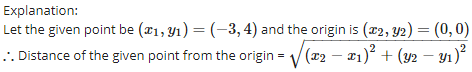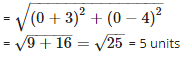Test: Coordinate Geometry- 3 - Question 2

### If A and B are the points ( – 6, 7) and ( – 1, – 5) respectively, then the distance 2AB is equal to

Detailed Solution for Test: Coordinate Geometry- 3 - Question 2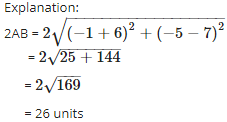Test: Coordinate Geometry- 3 - Question 3

### Three consecutive vertices of a parallelogram ABCD are A(1, 2), B(1, 0) and C(4, 0). The co – ordinates of the fourth vertex D are

Detailed Solution for Test: Coordinate Geometry- 3 - Question 3

Explanation:

Coordinates are given for  A(1 , 2) , B(1 , 0) and C(4 , 0)

Let coordinates of D be (x,y).
Since diagonals of a parallelogram bisect each other. at point O

Therefore O is the mid point of diagonal AC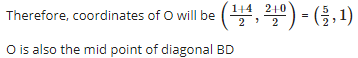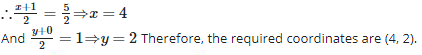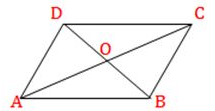Test: Coordinate Geometry- 3 - Question 4

The distance between the points ( – 1, – 5) and ( – 6, 7) is

Detailed Solution for Test: Coordinate Geometry- 3 - Question 4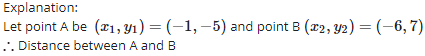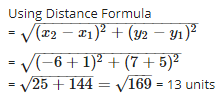Test: Coordinate Geometry- 3 - Question 5

The length of the median through A of  ΔABC with vertices A(7, – 3), B(5, 3) and C(3, – 1) is

Detailed Solution for Test: Coordinate Geometry- 3 - Question 5

Explanation:

ABC is a triangle with A(7 ,- 3), B(5 , 3) and C(3 , - 1)

Let median on BC bisects BC at D.          (AD is given as the median)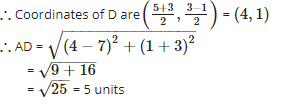Test: Coordinate Geometry- 3 - Question 6

If (a, 0) , (0, b) and (x, y) are collinear, then

Detailed Solution for Test: Coordinate Geometry- 3 - Question 6

Explanation:

If given points are collinear, the area of triangle formed by these three points is 0.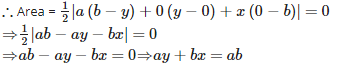Test: Coordinate Geometry- 3 - Question 7

If the distance between the points (p, – 5) and (2, 7) is 13 units, then the value of ‘p’ is

Detailed Solution for Test: Coordinate Geometry- 3 - Question 7

Explanation:

Let point A be (p,−5) and point B (2, 7) and distance between A and B = 13 units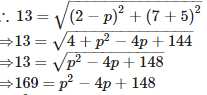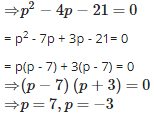Test: Coordinate Geometry- 3 - Question 8

The vertices of a quadrilateral are (1, 7), (4, 2), ( – 1, – 1) and ( – 4, 4). The quadrilateral is a

Detailed Solution for Test: Coordinate Geometry- 3 - Question 8

Explanation:

Let A (1, 7), B (4, 2), C(−1,−1) and D(−4,4) are the vertices of a quadrilateral ABCD.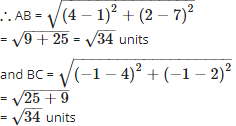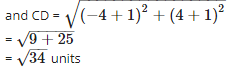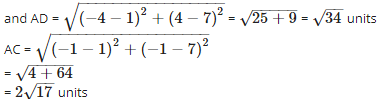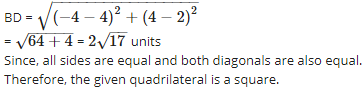Test: Coordinate Geometry- 3 - Question 9

The point ( – 3, 5) lies in the ___________ quadrant

Detailed Solution for Test: Coordinate Geometry- 3 - Question 9

Explanation:

Since x−coordinate is negative and y−coordinate is positive.Therefore, the point (−3,5) lies in II quadrant.

Test: Coordinate Geometry- 3 - Question 10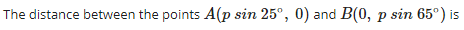Detailed Solution for Test: Coordinate Geometry- 3 - Question 10

The sum is according to distance formula
= √(x₂-x₁)²+(y₂-y₁)²
= √(0-p cos 25)² + (p cos 65-0)
= √(p cos 25)² + (p cos  65)²
= √(p cos 25)² + (p sin 25)²
= √(p² cos²25) + (a² sin²25)
= √p²(cos²25 + sin²25)                  we know that  sin²Θ + cos²Θ = 1
= √p²(1)
= √p²
= p Units

Test: Coordinate Geometry- 3 - Question 11

The base of an equilateral triangle ABC lies on the y – axis. The co – ordinates of the point C is (0, – 3). If origin is the midpoint of BC, then the co – ordinates of B are

Detailed Solution for Test: Coordinate Geometry- 3 - Question 11

Explanation:

Let the coordinate of B be (0,a).
It is given that (0, 0) is the mid-point of BC.

Therefore 0 = (0 + 0) /2 , 0 =(a - 3) /2   a - 3 = 0 ,  a = 3

Therefore, the coordinates of B are (0, 3).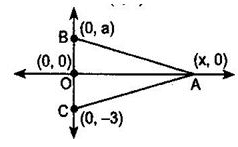Test: Coordinate Geometry- 3 - Question 12

If the co – ordinates of a point are (3, – 7), then its ordinate is

Detailed Solution for Test: Coordinate Geometry- 3 - Question 12

Explanation:

Since y−coordinate of a point is called ordinate.its distance from the x-axis measured parralel to the  y - axisTherefore, ordinate is −7.

Test: Coordinate Geometry- 3 - Question 13

The values of ‘y’ for which the distance between the points (2, – 3) and (10, y) is 10 units is

Detailed Solution for Test: Coordinate Geometry- 3 - Question 13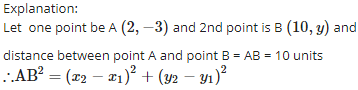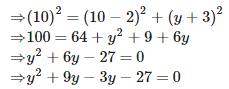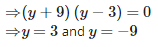Test: Coordinate Geometry- 3 - Question 14

If A is point on the x – axis whose abscissa is 5 and B is the point (1, – 3), then the distance AB is

Detailed Solution for Test: Coordinate Geometry- 3 - Question 14

Explanation:

Since A is a point on the x - axis, therefore coordinates of A are (5 , 0)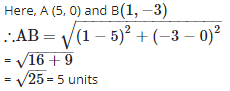Test: Coordinate Geometry- 3 - Question 15

The point where the perpendicular bisector of the line segment joining the points A(2, 5) and B(4, 7) cuts is:

Detailed Solution for Test: Coordinate Geometry- 3 - Question 15

Explanation:

Since, the point, where the perpendicular bisector of a line segment joining the points A(2 , 5) and B(4 , 7) cuts, is the mid-point of that line segment.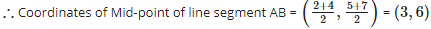## Quantitative Aptitude (Quant)

163 videos|152 docs|131 tests
 Use Code STAYHOME200 and get INR 200 additional OFF Use Coupon Code
Information about Test: Coordinate Geometry- 3 Page
In this test you can find the Exam questions for Test: Coordinate Geometry- 3 solved & explained in the simplest way possible. Besides giving Questions and answers for Test: Coordinate Geometry- 3, EduRev gives you an ample number of Online tests for practice

## Quantitative Aptitude (Quant)

163 videos|152 docs|131 tests